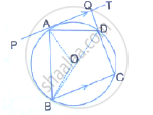Share

In the Given Figure, Pat is Tangent to Circle with Centre O at Point a on Its Circumference and is Parallel to Chord Bc. If Cdq is a Line Segment (I) ∠Bap = ∠Adq (Ii) ∠Aob = 2∠Adq (Iii) ∠Adq = ∠Adb - ICSE Class 10 - Mathematics

Question

In the given figure, PAT is tangent to the circle with centre O at point A on its circumference and is parallel to chord BC. If CDQ is a line segment , show that:
(i) ∠BAP = ∠ADQ

(ii) ∠AOB = 2∠ADQSolution

i) Since PAT ∥ BC

∴ ∠PAB = ∠ABC (alternate angles) .........(i)
In cyclic quadrilateral ABCD,
Ext ∠ADQ = ∠ABC ………..(ii)
From (i) and (ii)
∠PAB = ∠ADQ
ii) Arc AB subtends AOB at the centre and ADB at the remaining part of the circle.
∴ ∠AOB = 2∠ADB
⇒  ∠AOB = 2∠PAB (angles in alternate segments)
⇒ ∠AOB = 2∠ADQ (proved in (i) part)

iii)
∴  ∠BAP = ∠ADB (angles in alternate segments)
But
∠BAP = ∠ADQ (proved in (i) part)
∴  ∠ADQ = ∠ADB

Is there an error in this question or solution?

APPEARS IN

Solution In the Given Figure, Pat is Tangent to Circle with Centre O at Point a on Its Circumference and is Parallel to Chord Bc. If Cdq is a Line Segment (I) ∠Bap = ∠Adq (Ii) ∠Aob = 2∠Adq (Iii) ∠Adq = ∠Adb Concept: Cyclic Properties.
S